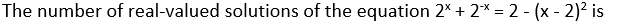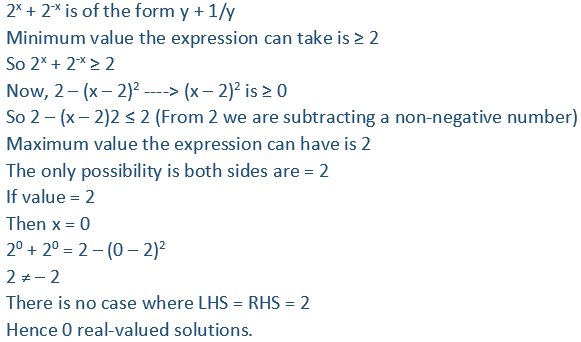# The number of real-valued solutions of the equation | Quantitative Aptitude - CAT Equation

## CAT 2020 - Slot 1 - Quantitative Aptitude - Equation - Question 6 - The number of real-valued solutions of the equation

Q. 6:##### 1. infinite2. 03. 14. 2### Past Year Question Paper & SolutionsCounselling Session
By IIM Mentor

#### Free Material Area